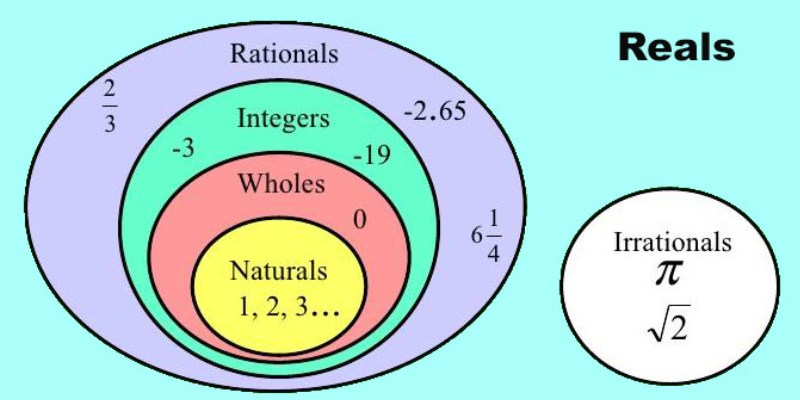# Ultimate Trivia Quiz On Real Numbers For 10th Grade

Questions : 11 | Total Attempts: 583 | Recent Updated: 13-May-2020A number is a mathematical thing that is used to count, measure, and label anything. There are different types of numbers like natural numbers, whole numbers, integers, and real numbers. Real numbers are those whose value is fixed and they are not imaginary. So take this quiz and know more about the real numbers.

### Questions Excerpt

1. Find the HCF of 1848, 3058, and 1331.

A. 9

B. 14

C. 13

D. 11

2. An army contingent of 616 members is to march behind an army band of 32 members in a parade. The two groups are to march in the same number of columns. What is the maximum number of columns in which they can march?

A. 8

B. 7

C. 6

D. 9

3. Let x= p/q be a rational number, such that the prime factorization of q is not of the form, where n, m are non-negative integers. Then, x has a decimal expansion which is ____________

A. Terminating

B. Non-terminating non-repeating

C. Terminating non-repeating

D. Non-terminating repeating (recurring)

4. Decide whether 52.123456789 is a rational number or not. If rational (in the form p/q), what can you say about the prime factors of q?

A. Rational Number, Prime factor of q will be only 2.

B. Rational Number, Prime factor of q will have a factor other than 2 or 5.

C. Not rational number.

D. Rational Number, Prime factors of q will have either 2 or 5 or both.

5. Without actually performing the long division, state whether the following rational numbers will have a terminating decimal expansion or a non-terminating decimal expansion: 23/8

A. Non-terminating non – repeating decimal

B. Terminating decimal

C. on-terminating repeating decimal

D. Option 4

6. Write down the decimal expansions of 13/ 6250

A. 0.0208

B. 0.00208

C. 0.00512

D. 0.00416

7. Which among the following options is irrational?

A. 3.1415926535

B. 10.2

C. -0.2

D. 0.2

8. Which one of the following can’t be the square of a natural number?

A. 20164

B. 42437

C. 81225

D. 32761

9. If the number 91876y2 is completely divisible by 8, then the smallest whole number in place of y will be:

A. 2

B. 4

C. 3

D. 1

10. a and b, when divided by 7 and 6 respectively, leave remainders p and q respectively. What is the maximum value of p + q?

A. 5

B. 6

C. 12

D. 11

11. The difference between the two numbers is 1365. On dividing the larger number by the smaller, we get 6 as quotient and the 15 as remainder. What is the smaller number?

A. 360

B. 295

C. 240

D. 270

## The Hundred Dresses Quiz

Wanda Petronski is the sole novice in her school with a 'funny' Polish call in "The Hundred Dresses," a tale of a female from a favela. The Hundred Dresses is primarily based totally on a real tale, as the writer describes having witnessed a comparab

## The Thief Story Quiz

The tale The Thief Story was written by Ruskin Bond. Ruskin Bond (born 19 May 1934) is an Indian writer. The tale is all about a teen thief who switches his name each month to live beforehand of the police and old employers. This time he holds on to,

## A Tiger in the Zoo Quiz: How Much You Know About A Tiger in the Zoo?

The poem written by Leslie Norris explains the agony and helplessness of a caged tiger that lives in a zoo. The poet explains what his life could be if he had been a free animal. The poet has tried to explain the condition of animals that are caged b

## Footprints Without Feet Trivia Quiz

In his piece Footprints Without Feet, the author, Herbert George Wells, explains how a scientist, Griffin abused his findings. The plot hinges around the scientist's creation of a rare invisibility substance. He created a medication that rendered him

## Dust Of Snow Poem Quiz For 10th Grade Students

There are millions of poems that we go through in our lives with and can relate to ourselves. Probably, some poems in our different grades have also been the same way. We know that we all go through the crossroads and challenges of life and see how o

## Quiz: How Much You Know About Metal And Non-Metal?

Metals are elements that are hard, a good conductor of heat and electricity, malleable, sonorous, lustrous and they have high melting and boiling point except for cesium and gallium. Whereas non-metal don’t show such properties they are brittle, non-